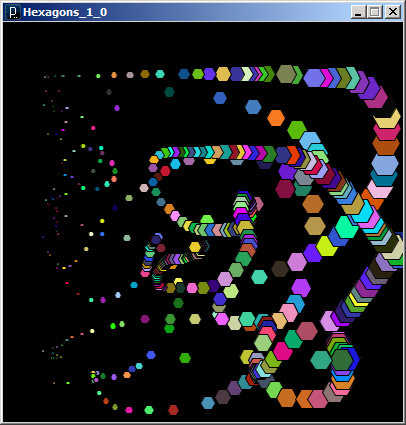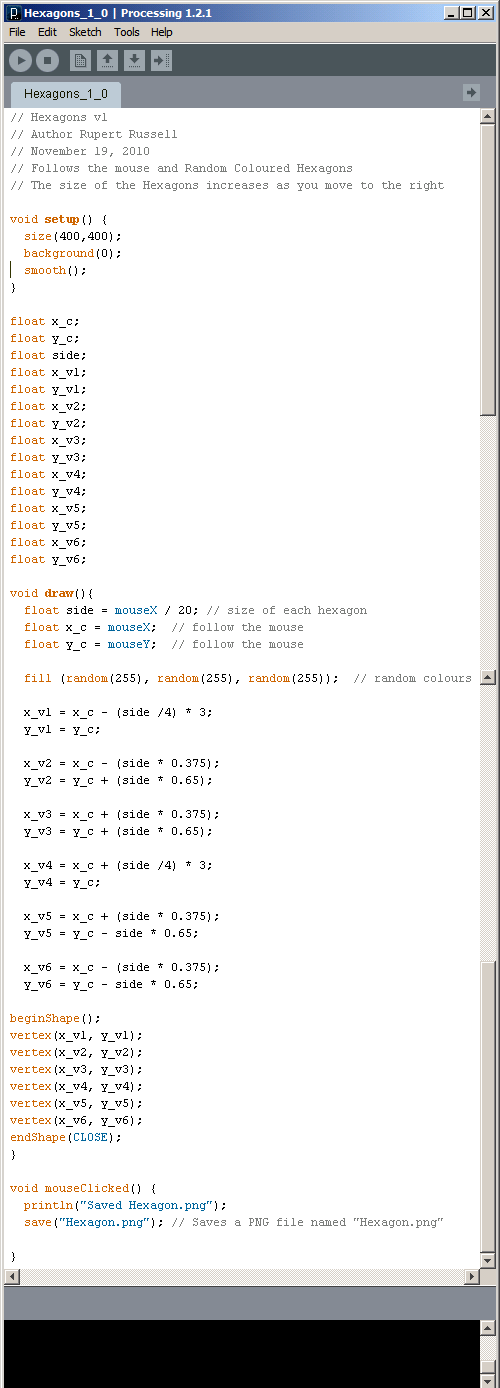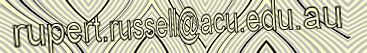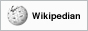Home > Tutorials > Processing > Processing Examples > Hexagons 1.0

# Hexagons 1.0

I had fun with this one, It draws randomly coloured hexagons using the mouse. It also scales the hexagons depending on the X value of the cursor. Try adding stroke(0,0,0,30); to get a different effect.#### processing code

```// Hexagons v1
// Author Rupert Russell
// November 19, 2010
// Follows the mouse and Random Coloured Hexagons
// The size of the Hexagons increases as you move to the right

void setup() {
size(400,400);
background(0);
smooth();
}

float x_c;
float y_c;
float side;
float x_v1;
float y_v1;
float x_v2;
float y_v2;
float x_v3;
float y_v3;
float x_v4;
float y_v4;
float x_v5;
float y_v5;
float x_v6;
float y_v6;

void draw(){
float side = mouseX / 20; // size of each hexagon
float x_c = mouseX;  // follow the mouse
float y_c = mouseY;  // follow the mouse

fill (random(255), random(255), random(255));  // random colours

x_v1 = x_c - (side /4) * 3;
y_v1 = y_c;

x_v2 = x_c - (side * 0.375);
y_v2 = y_c + (side * 0.65);

x_v3 = x_c + (side * 0.375);
y_v3 = y_c + (side * 0.65);

x_v4 = x_c + (side /4) * 3;
y_v4 = y_c;

x_v5 = x_c + (side * 0.375);
y_v5 = y_c - side * 0.65;

x_v6 = x_c - (side * 0.375);
y_v6 = y_c - side * 0.65;

beginShape();
vertex(x_v1, y_v1);
vertex(x_v2, y_v2);
vertex(x_v3, y_v3);
vertex(x_v4, y_v4);
vertex(x_v5, y_v5);
vertex(x_v6, y_v6);
endShape(CLOSE);
}

void mouseClicked() {
println("Saved Hexagon.png");
save("Hexagon.png"); // Saves a PNG file named "Hexagon.png"

}```

code formatter

APA citation:
Russell, R. (2016, July 05, 07:26 am). Hexagons 1.0
Retrieved June 07, 2023, from http://www.rupert.id.au/tutorials/processing/examples/hexagons_1/index.php

Last refreshed: June 07 2023. 04:21.54 pmThis work is licensed under a Creative Commons Attribution-NonCommercial-ShareAlike 2.5 License.

279 visits since November 19, 2010